Open in App
Not now

# Mathematical explanation for Linear Regression working

• Difficulty Level : Easy
• Last Updated : 18 Oct, 2022

Suppose we are given a dataset: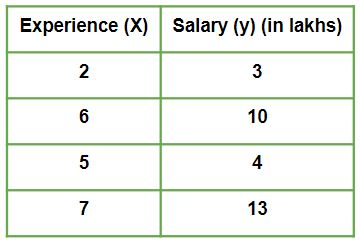Given is a Work vs Experience dataset of a company and the task is to predict the salary of a employee based on his / her work experience.
This article aims to explain how in reality Linear regression mathematically works when we use a pre-defined function to perform prediction task.
Let us explore how the stuff works when Linear Regression algorithm gets trained.

Iteration 1 – In the start, θ0 and θ1 values are randomly chosen. Let us suppose, θ0 = 0 and θ1 = 0.

• Predicted values after iteration 1 with Linear regression hypothesis.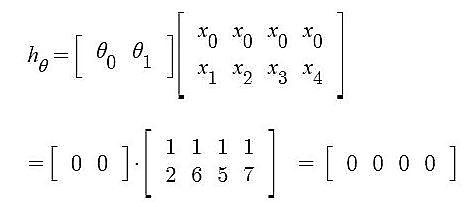• Cost Function – Error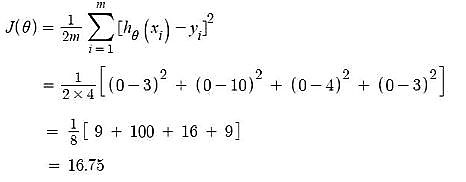• Gradient Descent – Updating θ0 value
Here, j = 0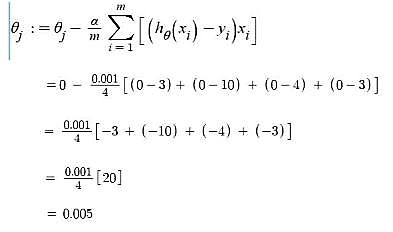• Gradient Descent – Updating θ1 value
Here, j = 1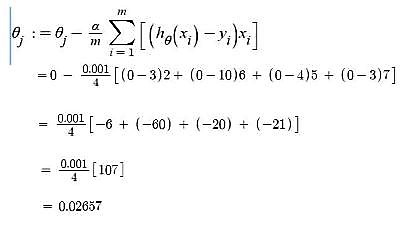Iteration 2 – θ0 = 0.005 and θ1 = 0.02657

• Predicted values after iteration 1 with Linear regression hypothesis.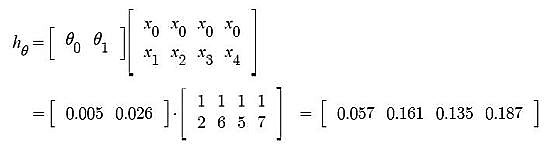Now, similar to iteration no. 1 performed above we will again calculate Cost function and update θj values using Gradient Descent.
We will keep on iterating until Cost function doesn’t reduce further. At that point, model achieves best θ values. Using these θ values in the model hypothesis will give the best prediction results.

My Personal Notes arrow_drop_up
Related Articles